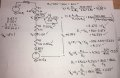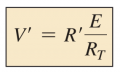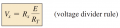# Circuit Analysis Problem

#### SamR

Joined Mar 19, 2019
4,818
Trying to understand this problem. Get most of it but the R4 stub and the opposing voltages are puzzling me. So, I put it into LTS and checked all the voltages which are annotated onto the LTS schematic. And my calculations agree.Analyzed and have no problem with the V1 to V2 part of the circuit. The R4 part is where I'm a bit confused. Just sitting there with R4 unattached there is 0V dropped across it because it is floating. I think I understand that. And when I measure the out tag I get the 46.33V that I see at V1 because there is no voltage dropped across R4? BUT! Am I not measuring that out tag voltage to ground? And IF I am measuring it ground that would mean that I am dropping all of the 46.33V across R4 even though I measure 0V across it floating and 46.33V at the out tag when it is floating? How can it be both 0V and 46.33V? In fact, I only have 20V from the 50V E1 being opposed by the 30V E2! Yes I understand that I am measuring from the 50V E1 to ground but that 50V is being opposed by the 30V from E2.

#### WBahn

Joined Mar 31, 2012
29,165
Yes, you are measuring the voltage relative to "ground" (really, relative to the reference node, which we sloppily call "ground").

You are dropping 46.33 V between the OUT tag and ground. You are also dropping 46.33 V between the V1 node and ground. That means that you are dropping 0 V between the OUT tag and the V1 node.

Remember, "dropping" just means the difference in voltage between two points.

Think of it like the height above the ground at various points in a building. Imagine a window in which the window sill is located 40 feet above the ground. Now you go to a different window and its sill is located 50 feet above the ground. That's all you need to know to figure out that the second window is 10 feet higher than the first window.

So what if the second window is located 40 feet above ground? That means that the two windows are at the same height such that there is zero difference between the height of the two windows.

It's the same with your circuit. V1 is 46.33 V above ground and so is OUT. That means there this no voltage difference between those two points (which IS the voltage across R4).

•SamR

#### boostbuck

Joined Oct 5, 2017
381
.....IF I am measuring it ground that would mean that I am dropping all of the 46.33V across R4.....
No, you are dropping all of the 46.33V across the measuring instrument, because it has a resistance much larger than the resistances in the circuit.

#### SamR

Joined Mar 19, 2019
4,818
In this case that isn't quite true as I am using an LTS simulator instead of an actual multimeter.

#### ericgibbs

Joined Jan 29, 2010
18,032
Hi Sam,
I would just a Current analysis.
You have 50v-30v acting across a 18k resistance. ie 1.11' mA.
So across the 3k3 there is a 3.66' Vdrop.
So the junction is at 50v -3.66v = 46.33'v
E

#### WBahn

Joined Mar 31, 2012
29,165
In this case that isn't quite true as I am using an LTS simulator instead of an actual multimeter.
It's even more true, then. The resistance of the simulator's voltmeter is infinite.

•boostbuck and SamR